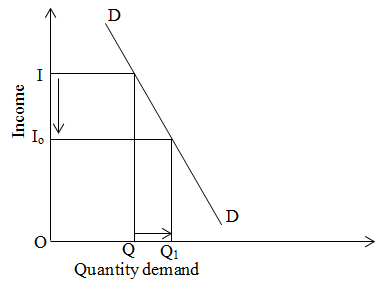# Negative income elasticity of demand

If there is negative relationship between income and demand in this case income elasticity is negative. In this case, inferior goods income elasticity is negative.

We can explain it by the given figure:On the above figure, x and y axis represent demand for inferior goods and income respectively. When income is OI then quantity demand is OQ and when income decreases from I to Io then quantity demand increases from Q to Q1.